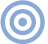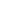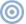# Reference

## Audio Basics: A Is For Ampere Page 5

An alternative way of expressing that transition frequency is as a charge/discharge time constant, in microseconds (µs). The time constant is calculated by multiplying the resistance by the capacitance. To convert from time constant to frequency, or vice versa, just use the magic 160,000. Divide the known quantity into it, and that's it.

Another interesting property of a charged capacitor is that it produces what's called an electrostatic field, in which the negative energy from packed electrons actually radiates for some distance from the negatively charged plate, and will attract anything that has a less negative (or a more positive) charge. This is "interesting" to audiophiles only because it is the basis of electrostatic loudspeakers.

Inductors
This is electronics argot for a coil of wire, and the term inductance (symbol L) is used to describe its electrical and magnetic properties, some of which are almost perfectly complementary to those of a capacitor. Instead of generating an electrostatic field, an inductor produces a magnetic field when current passes through it. Instead of blocking DC, it passes as much as its electrical resistance will permit. And instead of passing high frequencies more readily than low, its impedance increases with increasing frequency.

When DC is first passed through the coil, the current builds a magnetic field through the center of it, taking a brief but finite time to do it. In fact, its rise to full magnetism looks exactly like the charge curve of a capacitor. When the DC is removed, the collapse of the field follows the capacitor's discharge curve. But they differ in one other important aspect: phase. (In this context, this much-misunderstood word fundamentally describes the time relationship between the voltage sinewave and the current sinewave.) With a capacitor, the charging current is always slightly ahead in time of the voltage developed by it. In an inductor, though, its own magnetic field acts increasingly to resist current flow through the coil, so the current is always lagging slightly behind the voltage that is across the coil. (Physicists call this self-inductance, but what do they know?)

The faster the current changes, the more the inductor resists it. That is, its series impedance (Remember? AC resistance?) is highest at high frequencies and lowest at low frequencies. The more turns of wire the coil has, the higher the inductance and the greater opposition it has to AC passing through it. Its state of maximum magnetic field is analogous (but complementary) to a capacitor's full charge state. In the case of the capacitor, no more current flows; in the case of an inductor, as much current flows as the coil's DC resistance will allow. At low alternating frequencies, with time enough between alternations for the magnetic field to build up fully, the effect (impedance) of the coil diminishes until, eventually, only the DC resistance of its wire is in the circuit. Thus, resistors and inductors can be combined (in an LR network) to do things which are complementary to what RC networks can do.

Combinations of L and C behave quite differently from either one in combination with a resistor; their complementary properties interact to produce impedance variations which are much greater, and much sharper with changing frequency, than those achievable by either element alone. (This has to do with the fact that one's current leads while the other's lags, but that's too complicated to get into. Forget I mentioned it.) LC filters are used where very steep attenuation curves are needed, or whenever it is necessary to produce a deep, narrow dip or peak in the frequency response. LC combinations are used as bandpass filters in multi-band equalizers, and for selecting radio broadcast stations.

Inductors are not often used in high-end audio circuits, but are sometimes essential, as when it is necessary (for whatever reason) to isolate the output of a power amplifier from its load at high frequencies, or to reduce the 60Hz series impedance of a power supply, so that a given value of filter capacitance (between the supply and ground) will divide-out more leftover AC ripple from the rectifier's outputs. Inductors are also the basis of a device which is almost universally used in audio: the transformer.ARTICLE CONTENTSX